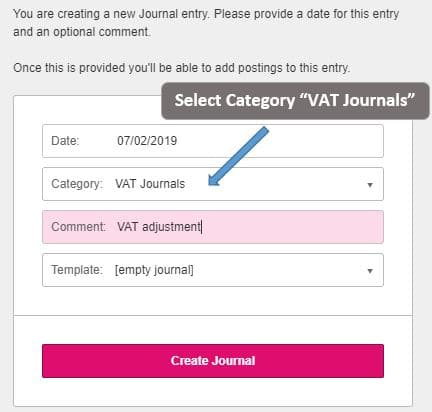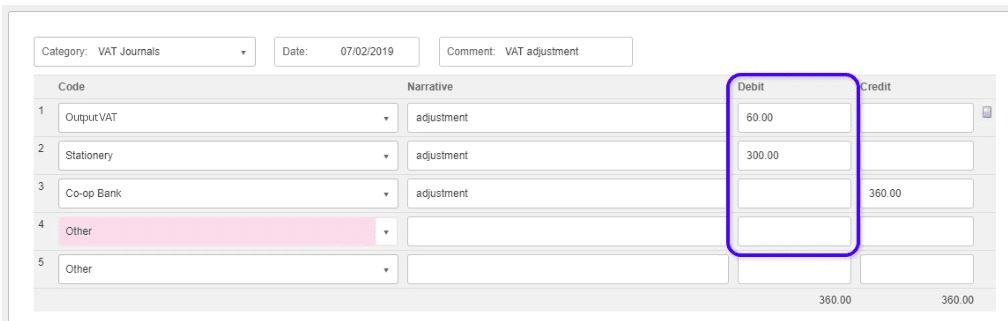# How to amend box values on VAT return using a journal

You are able to amend the boxes on the VAT return by doing a VAT journal.

You would need to follow the below steps:

Go to Journals> + Create and select the category of “VAT Journal”You can only create a journal for Input VAT or Output VAT adjustments separately, if you need to update both areas then you will need to create two journals.

When you create the journal and enter the line for Input VAT or Output VAT, any other nominal’s on that journal which have a value on the same debit or debit side will be updated on the VAT return.

In the below example, as Output VAT is entered on the Debit only.  Only other nominal lines on that journal that are on the debit side also will appear on the VAT return.This will update the VAT Return for the following boxes:

• Box 1 = 60.00
• Box 6 = 300.00

Below are other examples of how to enter the journal and which boxes will be effected on the VAT return.

Output VAT 1

CodeDebitCredit
Output VAT20.00
Net for VAT Return Code100.00
Balancing Code120.00

EC Member State for VAT Return = Un-ticked

• VAT Return
• Box 1 = 20.00
• Box 6 = 100.00

Output VAT 2

CodeDebitCredit
Output VAT20.00
Net for VAT Return Code100.00
Balancing Code120.00

EC Member State for VAT Return = Un-ticked

• VAT Return
• Box 1 = -20.00
• Box 6 = -100.00

Output VAT 3

CodeDebitCredit
Output VAT20.00
Net for VAT Return Code100.00
Output VAT5.00
Net for VAT Return Code100.00
Balancing Code225.00

EC Member State for VAT Return = Un-ticked

• VAT Return
• Box 1 = 25.00
• Box 6 = 200.00

Output VAT 1 with EC Member State for VAT Return selected

CodeDebitCredit
Output VAT20.00
Net for VAT Return Code100.00
Balancing Code120.00

EC Member State for VAT Return = Ticked

• VAT Return
• Box 1 = 20.00
• Box 6 = 100.00
• Box 8 = 100.00

Output VAT 2 with EC Member State for VAT Return selected

CodeDebitCredit
Output VAT20.00
Net for VAT Return Code100.00
Balancing Code120.00

EC Member State for VAT Return = Ticked

• VAT Return
• Box 1 = -20.00
• Box 6 = -100.00
• Box 8 = -100.00

Output VAT 3 with EC Member State for VAT Return selected

CodeDebitCredit
Output VAT20.00
Net for VAT Return Code100.00
Output VAT5.00
Net for VAT Return Code100.00
Balancing Code225.00

EC Member State for VAT Return = Ticked

• VAT Return
• Box 1 = 25.00
• Box 6 = 200.00
• Box 8 = 200.00

Input VAT 1

CodeDebitCredit
Input VAT20.00
Net for VAT Return Code100.00
Balancing Code120.00

EC Member State for VAT Return = Un-ticked

• VAT Return
• Box 4 = 20.00
• Box 7 = 100.00

Input VAT 2

CodeDebitCredit
Input VAT20.00
Net for VAT Return Code100.00
Balancing Code120.00

EC Member State for VAT Return = Un-ticked

• VAT Return
• Box 4 = -20.00
• Box 7 = -100.00

Input VAT 3

CodeDebitCredit
Input VAT20.00
Net for VAT Return Code100.00
Input VAT5.00
Net for VAT Return Code100.00
Balancing Code225.00

EC Member State for VAT Return = Un-ticked

• VAT Return
• Box 4 = 25.00
• Box 7 = 200.00

Input VAT 1 with EC Member State for VAT Return selected

CodeDebitCredit
Input VAT20.00
Net for VAT Return Code100.00
Balancing Code120.00

EC Member State for VAT Return = Ticked

• VAT Return
• Box 2 = 20.00
• Box 4 = 20.00
• Box 7 = 100.00
• Box 9 = 100.00

Input VAT 2 with EC Member State for VAT Return selected

CodeDebitCredit
Input VAT20.00
Net for VAT Return Code100.00
Balancing Code120.00

EC Member State for VAT Return = Ticked

• VAT Return
• Box 2 = -20.00
• Box 4 = -20.00
• Box 7 = -100.00
• Box 9 = -100.00

Input VAT 3 with EC Member State for VAT Return selected

CodeDebitCredit
Input VAT20.00
Net for VAT Return Code100.00
Input VAT5.00
Net for VAT Return Code100.00
Balancing Code225.00

EC Member State for VAT Return = Ticked

• VAT Return
• Box 2 = 25.00
• Box 4 = 25.00
• Box 7 = 200.00
• Box 9 = 200.00